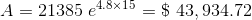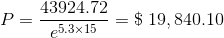Homework Help Question & Answers

(1 point) Your rich uncle the bequest require that this income stream be paid continuously into a...(1 point) Your rich uncle the bequest require that this income stream be paid continuously into a specific savings account that will not be available to you for 15 years. This account earns 4.8% interest, compounded continuously. to you a continuous, constant income stream of \$2000 per year for the next 15 years. The terms of What is the present value of the bequest? 21385.322 How much money would the bequest be worth (including all interest accrued) after 15 years? You discover that a bank is What is the cost of a CD at the above interest rate that would provide the same amount of money as the bequest after 15 offering 5.3% interest compounded continuously on a certificate of deposit (CD) that matures in 15 years. You ask the executor of the estate to buy a CD now whose value after 15 years will be the same as the amount that would be available to you in 15 years under the origional terms of the bequest, and to pay you the difference between the present value of the original bequest and the amount invested in the CD. How much do you get now? Note: You can earn partial credit on this problem.

Continuous Compounding Formulas (n → ∞)

To calculate Accrued Amount (Principal + interest) :where P is the principal Amount and r- rate of interest and t the time .

The Bequest will be worth (after 15 years)

P = 21385.322, r = 4.8 % and t = 15 substituting into equation (1)

we getfor the CD, we need A= \$ 43,934.92 in 15 years (@ 5.3% interest rate). we need to find the principal:

rewriting equation (1) in terms of P:thereforeProfit made = 21385.322 - 19840.10 = \$ 1545.222.

Add Answer of: (1 point) Your rich uncle the bequest require that this income stream be paid continuously into a...
More Homework Help Questions Additional questions in this topic.

• Find the approximate (with an integral estimate) present and future values of a constant income stream of 2500 dollars per year over a period of 40 years, assume a 5 percent annual interest rate compounded continuously

Need Online Homework Help?

Get FREE EXPERT Answers
WITHIN MINUTES
Related Questions